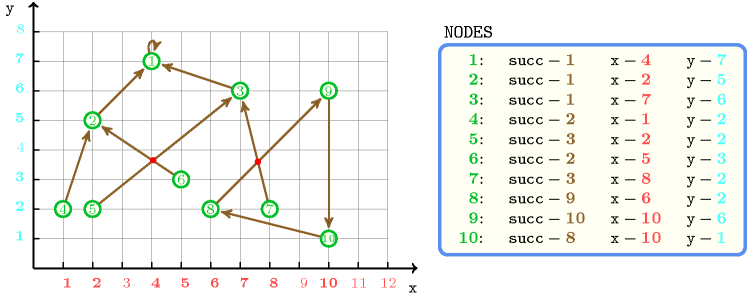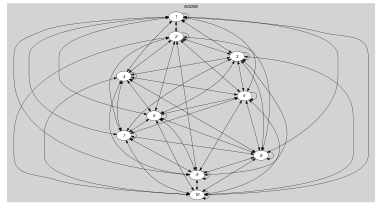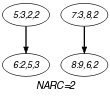## 5.170. graph_crossing

Origin

N. Beldiceanu

Constraint

$\mathrm{𝚐𝚛𝚊𝚙𝚑}_\mathrm{𝚌𝚛𝚘𝚜𝚜𝚒𝚗𝚐}\left(\mathrm{𝙽𝙲𝚁𝙾𝚂𝚂},\mathrm{𝙽𝙾𝙳𝙴𝚂}\right)$

Synonyms

$\mathrm{𝚌𝚛𝚘𝚜𝚜𝚒𝚗𝚐}$, $\mathrm{𝚗𝚌𝚛𝚘𝚜𝚜}$.

Arguments
 $\mathrm{𝙽𝙲𝚁𝙾𝚂𝚂}$ $\mathrm{𝚍𝚟𝚊𝚛}$ $\mathrm{𝙽𝙾𝙳𝙴𝚂}$ $\mathrm{𝚌𝚘𝚕𝚕𝚎𝚌𝚝𝚒𝚘𝚗}\left(\mathrm{𝚜𝚞𝚌𝚌}-\mathrm{𝚍𝚟𝚊𝚛},𝚡-\mathrm{𝚒𝚗𝚝},𝚢-\mathrm{𝚒𝚗𝚝}\right)$
Restrictions
 $\mathrm{𝙽𝙲𝚁𝙾𝚂𝚂}\ge 0$ $\mathrm{𝚛𝚎𝚚𝚞𝚒𝚛𝚎𝚍}$$\left(\mathrm{𝙽𝙾𝙳𝙴𝚂},\left[\mathrm{𝚜𝚞𝚌𝚌},𝚡,𝚢\right]\right)$ $\mathrm{𝙽𝙾𝙳𝙴𝚂}.\mathrm{𝚜𝚞𝚌𝚌}\ge 1$ $\mathrm{𝙽𝙾𝙳𝙴𝚂}.\mathrm{𝚜𝚞𝚌𝚌}\le |\mathrm{𝙽𝙾𝙳𝙴𝚂}|$
Purpose

$\mathrm{𝙽𝙲𝚁𝙾𝚂𝚂}$ is the number of proper intersections between line segments, where each line segment is an arc of the directed graph defined by the arc linking a node and its unique successor.

Example
$\left(\begin{array}{c}2,〈\begin{array}{ccc}\mathrm{𝚜𝚞𝚌𝚌}-1\hfill & 𝚡-4\hfill & 𝚢-7,\hfill \\ \mathrm{𝚜𝚞𝚌𝚌}-1\hfill & 𝚡-2\hfill & 𝚢-5,\hfill \\ \mathrm{𝚜𝚞𝚌𝚌}-1\hfill & 𝚡-7\hfill & 𝚢-6,\hfill \\ \mathrm{𝚜𝚞𝚌𝚌}-2\hfill & 𝚡-1\hfill & 𝚢-2,\hfill \\ \mathrm{𝚜𝚞𝚌𝚌}-3\hfill & 𝚡-2\hfill & 𝚢-2,\hfill \\ \mathrm{𝚜𝚞𝚌𝚌}-2\hfill & 𝚡-5\hfill & 𝚢-3,\hfill \\ \mathrm{𝚜𝚞𝚌𝚌}-3\hfill & 𝚡-8\hfill & 𝚢-2,\hfill \\ \mathrm{𝚜𝚞𝚌𝚌}-9\hfill & 𝚡-6\hfill & 𝚢-2,\hfill \\ \mathrm{𝚜𝚞𝚌𝚌}-10\hfill & 𝚡-10\hfill & 𝚢-6,\hfill \\ \mathrm{𝚜𝚞𝚌𝚌}-8\hfill & 𝚡-10\hfill & 𝚢-1\hfill \end{array}〉\hfill \end{array}\right)$

Figure 5.170.1 shows the line segments associated with the $\mathrm{𝙽𝙾𝙳𝙴𝚂}$ collection. One can note the following line segments intersection:

• Arcs $8\to 9$ and $7\to 3$ cross,

• Arcs $5\to 3$ and $6\to 2$ cross also.

Consequently, the $\mathrm{𝚐𝚛𝚊𝚙𝚑}_\mathrm{𝚌𝚛𝚘𝚜𝚜𝚒𝚗𝚐}$ constraint holds since its first argument $\mathrm{𝙽𝙲𝚁𝙾𝚂𝚂}$ is set to 2.

##### Figure 5.170.1. Illustration of the Example slot: a graph covering with 2 line segments intersections in red ($\mathrm{𝙽𝙲𝚁𝙾𝚂𝚂}=2$)Typical
 $|\mathrm{𝙽𝙾𝙳𝙴𝚂}|>1$ $\mathrm{𝚛𝚊𝚗𝚐𝚎}$$\left(\mathrm{𝙽𝙾𝙳𝙴𝚂}.\mathrm{𝚜𝚞𝚌𝚌}\right)>1$ $\mathrm{𝚛𝚊𝚗𝚐𝚎}$$\left(\mathrm{𝙽𝙾𝙳𝙴𝚂}.𝚡\right)>1$ $\mathrm{𝚛𝚊𝚗𝚐𝚎}$$\left(\mathrm{𝙽𝙾𝙳𝙴𝚂}.𝚢\right)>1$
Symmetries
• Attributes of $\mathrm{𝙽𝙾𝙳𝙴𝚂}$ are permutable w.r.t. permutation $\left(\mathrm{𝚜𝚞𝚌𝚌}\right)$ $\left(𝚡,𝚢\right)$ (permutation applied to all items).

• One and the same constant can be added to the $𝚡$ attribute of all items of $\mathrm{𝙽𝙾𝙳𝙴𝚂}$.

• One and the same constant can be added to the $𝚢$ attribute of all items of $\mathrm{𝙽𝙾𝙳𝙴𝚂}$.

Arg. properties

Functional dependency: $\mathrm{𝙽𝙲𝚁𝙾𝚂𝚂}$ determined by $\mathrm{𝙽𝙾𝙳𝙴𝚂}$.

Usage

This is a general crossing constraint that can be used in conjunction with one graph covering constraint such as $\mathrm{𝚌𝚢𝚌𝚕𝚎}$, $\mathrm{𝚝𝚛𝚎𝚎}$ or $\mathrm{𝚖𝚊𝚙}$. In many practical problems ones want not only to cover a graph with specific patterns but also to avoid too much crossing between the arcs of the final graph.

Remark

We did not give a specific crossing constraint for each graph covering constraint. We feel that it is better to start first with a more general constraint before going in the specificity of the pattern that is used for covering the graph.

Keywords
Arc input(s)

$\mathrm{𝙽𝙾𝙳𝙴𝚂}$

Arc generator
$\mathrm{𝐶𝐿𝐼𝑄𝑈𝐸}$$\left(<\right)↦\mathrm{𝚌𝚘𝚕𝚕𝚎𝚌𝚝𝚒𝚘𝚗}\left(𝚗\mathtt{1},𝚗\mathtt{2}\right)$

Arc arity
Arc constraint(s)
 $•\begin{array}{c}\mathrm{𝚖𝚊𝚡}\left(𝚗\mathtt{1}.𝚡,\mathrm{𝙽𝙾𝙳𝙴𝚂}\left[𝚗\mathtt{1}.\mathrm{𝚜𝚞𝚌𝚌}\right].𝚡\right)\ge \hfill \\ \mathrm{𝚖𝚒𝚗}\left(𝚗\mathtt{2}.𝚡,\mathrm{𝙽𝙾𝙳𝙴𝚂}\left[𝚗\mathtt{2}.\mathrm{𝚜𝚞𝚌𝚌}\right].𝚡\right)\hfill \end{array}$ $•\begin{array}{c}\mathrm{𝚖𝚊𝚡}\left(𝚗\mathtt{2}.𝚡,\mathrm{𝙽𝙾𝙳𝙴𝚂}\left[𝚗\mathtt{2}.\mathrm{𝚜𝚞𝚌𝚌}\right].𝚡\right)\ge \hfill \\ \mathrm{𝚖𝚒𝚗}\left(𝚗\mathtt{1}.𝚡,\mathrm{𝙽𝙾𝙳𝙴𝚂}\left[𝚗\mathtt{1}.\mathrm{𝚜𝚞𝚌𝚌}\right].𝚡\right)\hfill \end{array}$ $•\begin{array}{c}\mathrm{𝚖𝚊𝚡}\left(𝚗\mathtt{1}.𝚢,\mathrm{𝙽𝙾𝙳𝙴𝚂}\left[𝚗\mathtt{1}.\mathrm{𝚜𝚞𝚌𝚌}\right].𝚢\right)\ge \hfill \\ \mathrm{𝚖𝚒𝚗}\left(𝚗\mathtt{2}.𝚢,\mathrm{𝙽𝙾𝙳𝙴𝚂}\left[𝚗\mathtt{2}.\mathrm{𝚜𝚞𝚌𝚌}\right].𝚢\right)\hfill \end{array}$ $•\begin{array}{c}\mathrm{𝚖𝚊𝚡}\left(𝚗\mathtt{2}.𝚢,\mathrm{𝙽𝙾𝙳𝙴𝚂}\left[𝚗\mathtt{2}.\mathrm{𝚜𝚞𝚌𝚌}\right].𝚢\right)\ge \hfill \\ \mathrm{𝚖𝚒𝚗}\left(𝚗\mathtt{1}.𝚢,\mathrm{𝙽𝙾𝙳𝙴𝚂}\left[𝚗\mathtt{1}.\mathrm{𝚜𝚞𝚌𝚌}\right].𝚢\right)\hfill \end{array}$ $•\begin{array}{c}\left(𝚗\mathtt{2}.𝚡-\mathrm{𝙽𝙾𝙳𝙴𝚂}\left[𝚗\mathtt{1}.\mathrm{𝚜𝚞𝚌𝚌}\right].𝚡\right)*\left(\begin{array}{c}\mathrm{𝙽𝙾𝙳𝙴𝚂}\left[𝚗\mathtt{1}.\mathrm{𝚜𝚞𝚌𝚌}\right].𝚢-\hfill \\ 𝚗\mathtt{1}.𝚢\hfill \end{array}\right)-\hfill \\ \left(\mathrm{𝙽𝙾𝙳𝙴𝚂}\left[𝚗\mathtt{1}.\mathrm{𝚜𝚞𝚌𝚌}\right].𝚡-𝚗\mathtt{1}.𝚡\right)*\left(\begin{array}{c}𝚗\mathtt{2}.𝚢-\hfill \\ \mathrm{𝙽𝙾𝙳𝙴𝚂}\left[𝚗\mathtt{1}.\mathrm{𝚜𝚞𝚌𝚌}\right].𝚢\hfill \end{array}\right)\hfill \end{array}\ne 0$ $•\begin{array}{c}\left(\mathrm{𝙽𝙾𝙳𝙴𝚂}\left[𝚗\mathtt{2}.\mathrm{𝚜𝚞𝚌𝚌}\right].𝚡-\mathrm{𝙽𝙾𝙳𝙴𝚂}\left[𝚗\mathtt{1}.\mathrm{𝚜𝚞𝚌𝚌}\right].𝚡\right)*\left(\begin{array}{c}𝚗\mathtt{2}.𝚢-\hfill \\ 𝚗\mathtt{1}.𝚢\hfill \end{array}\right)-\hfill \\ \left(𝚗\mathtt{2}.𝚡-𝚗\mathtt{1}.𝚡\right)*\left(\begin{array}{c}\mathrm{𝙽𝙾𝙳𝙴𝚂}\left[𝚗\mathtt{2}.\mathrm{𝚜𝚞𝚌𝚌}\right].𝚢-\hfill \\ \mathrm{𝙽𝙾𝙳𝙴𝚂}\left[𝚗\mathtt{1}.\mathrm{𝚜𝚞𝚌𝚌}\right].𝚢\hfill \end{array}\right)\hfill \end{array}\ne 0$ $•\begin{array}{c}\mathrm{𝚜𝚒𝚐𝚗}\left(\begin{array}{c}\begin{array}{c}\prod \left(\begin{array}{c}𝚗\mathtt{2}.𝚡-\mathrm{𝙽𝙾𝙳𝙴𝚂}\left[𝚗\mathtt{1}.\mathrm{𝚜𝚞𝚌𝚌}\right].𝚡,\hfill \\ \mathrm{𝙽𝙾𝙳𝙴𝚂}\left[𝚗\mathtt{1}.\mathrm{𝚜𝚞𝚌𝚌}\right].𝚢-𝚗\mathtt{1}.𝚢\hfill \end{array}\right)-\hfill \\ \prod \left(\begin{array}{c}\mathrm{𝙽𝙾𝙳𝙴𝚂}\left[𝚗\mathtt{1}.\mathrm{𝚜𝚞𝚌𝚌}\right].𝚡-𝚗\mathtt{1}.𝚡,\hfill \\ 𝚗\mathtt{2}.𝚢-\mathrm{𝙽𝙾𝙳𝙴𝚂}\left[𝚗\mathtt{1}.\mathrm{𝚜𝚞𝚌𝚌}\right].𝚢\hfill \end{array}\right)\hfill \end{array}\hfill \end{array}\right)\ne \hfill \\ \mathrm{𝚜𝚒𝚐𝚗}\left(\begin{array}{c}\begin{array}{c}\prod \left(\begin{array}{c}\mathrm{𝙽𝙾𝙳𝙴𝚂}\left[𝚗\mathtt{2}.\mathrm{𝚜𝚞𝚌𝚌}\right].𝚡-\mathrm{𝙽𝙾𝙳𝙴𝚂}\left[𝚗\mathtt{1}.\mathrm{𝚜𝚞𝚌𝚌}\right].𝚡,\hfill \\ 𝚗\mathtt{2}.𝚢-𝚗\mathtt{1}.𝚢\hfill \end{array}\right)-\hfill \\ \prod \left(\begin{array}{c}𝚗\mathtt{2}.𝚡-𝚗\mathtt{1}.𝚡,\hfill \\ \mathrm{𝙽𝙾𝙳𝙴𝚂}\left[𝚗\mathtt{2}.\mathrm{𝚜𝚞𝚌𝚌}\right].𝚢-\mathrm{𝙽𝙾𝙳𝙴𝚂}\left[𝚗\mathtt{1}.\mathrm{𝚜𝚞𝚌𝚌}\right].𝚢\hfill \end{array}\right)\hfill \end{array}\hfill \end{array}\right)\hfill \end{array}$
Graph property(ies)
$\mathrm{𝐍𝐀𝐑𝐂}$$=\mathrm{𝙽𝙲𝚁𝙾𝚂𝚂}$

Graph model

Each node is described by its coordinates $𝚡$ and $𝚢$, and by its successor $\mathrm{𝚜𝚞𝚌𝚌}$ in the final covering. Note that the co-ordinates are initially fixed. We use the arc generator $\mathrm{𝐶𝐿𝐼𝑄𝑈𝐸}\left(<\right)$ in order to avoid counting twice the same line segment crossing.

Parts (A) and (B) of Figure 5.170.2 respectively show the initial and final graph associated with the Example slot. Since we use the $\mathrm{𝐍𝐀𝐑𝐂}$ graph property, the arcs of the final graph are stressed in bold. Each arc of the final graph corresponds to a proper intersection between two line segments.

##### Figure 5.170.2. Initial and final graph of the $\mathrm{𝚐𝚛𝚊𝚙𝚑}_\mathrm{𝚌𝚛𝚘𝚜𝚜𝚒𝚗𝚐}$ constraint(a)(b)